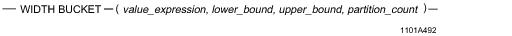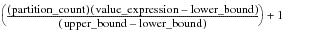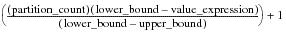# 15.00 - WIDTH_BUCKET - Teradata Database

## Teradata Database SQL Functions, Operators, Expressions, and Predicates

Product
Release Number
15.00
Content Type
Programming Reference
Publication ID
B035-1145-015K
Language
English (United States)

## WIDTH_BUCKET

### Purpose

Returns the number of the partition to which value_expression is assigned.

### Syntaxwhere:

 Syntax element … Specifies the … value_expression value for which a partition number is to be returned. lower_bound lower boundary for the range of values to be partitioned equally. upper_bound upper boundary for the range of values to be partitioned equally. partition_count number of partitions to be created. This value also specifies the width of the partitions by default. The number of partitions created is partition_count + 2. Partition 0 and partition partition_count + 1 account for values of value_expression that are outside the lower and upper boundaries.

### ANSI Compliance

This is a Teradata extension to the ANSI SQL:2011 standard.

### Result Type and Attributes

The data type, format, and title for WIDTH_BUCKET(x, l, u, y) are as follows.

 Data Type Format Title INTEGER the default format for INTEGER Width_bucket(x, l, u, y)

For information on default data type formats, see SQL Data Types and Literals.

### Argument Types and Rules

Use the following table for rules concerning WIDTH_BUCKET arguments.

 Data Type Rules Numeric WIDTH_BUCKET accepts all numeric data types as arguments. The arguments value_expression, lower_bound, and upper_bound are converted to REAL before processing. The partition_count argument is converted to INTEGER before processing. Character WIDTH_BUCKET accepts character strings that represent numeric values, and converts them to the appropriate numeric type. TIME, TIMESTAMP, or Period INTERVAL BYTE or VARBYTE BLOB or CLOB CHARACTER or VARCHAR if the server character set is GRAPHIC WIDTH_BUCKET does not accept these types of arguments. UDT The UDT must have an implicit cast to any of the following predefined types: Numeric Character DATE To define an implicit cast for a UDT, use the CREATE CAST statement and specify the AS ASSIGNMENT clause. For more information on CREATE CAST, see SQL Data Definition Language. Implicit type conversion of UDTs for system operators and functions, including WIDTH_BUCKET, is a Teradata extension to the ANSI SQL standard. To disable this extension, set the DisableUDTImplCastForSysFuncOp field of the DBS Control Record to TRUE. For details, see Utilities: Volume 1 (A-K).

If an argument cannot be implicitly converted to an acceptable type, an error is reported. For more information on implicit type conversion, see “Implicit Type Conversions” on page 583.

### Rules

The following rules apply to WIDTH_BUCKET:

• If any argument is null, then the result is also null.
• If partition_count <=0 or if partition_count > 2147483646, an error is returned to the requestor.
• If lower_bound = upper_bound, an error is returned to the requestor.
• If lower_bound < upper_bound, then the rules in the following table apply.
•
 IF … THEN the result is … value_expression < lower_bound 0. value_expression >= upper_bound partition_count +1. If the result cannot be represented by the data type specified for the result, then an error is returned. anything else the greatest exact numeric value with scale 0 that is less than or equal to the following expression.• If lower_bound > upper_bound, then the rules in the following table apply.
•
 IF … THEN the result is … value_expression > lower_bound 0. value_expression <= upper_bound partition_count +1. If the result cannot be represented by the data type specified for the result, then an error is returned. anything else the least exact numeric value with scale 0 that is less than or equal to the following expression.### Example

You want to create a histogram for the salaries of all employees whose salary amount ranges between \$70000 and \$200000. The width of each partition, or bucket, within the specified range is to be \$32500.

The employee salary table contains eight employees:

`salary   first_name   last_name`
`-------- ------------ -----------`
`50000    William      Crawford`
`150000   Todd         Crawford`
`220000   Bob          Stone`
`199999   Donald       Stone`
`70000    Betty        Crawford`
`70000    James        Crawford`
`70000    Mary         Lee`
`120000   Mary         Stone`

You perform the following SELECT statement.

`   SELECT salary, WIDTH_BUCKET(salary,70000,200000,4),COUNT(salary)`
`   FROM emp_salary`
`   GROUP BY 1`
`   ORDER BY 1;`

The report produced by this statement looks like this.

`salary   Width_bucket(salary,70000,200000,4)  Count(salary)`
`-------- ------------------------------------ ----------------`
`50000    0                                    1`
`70000    1                                    3`
`120000   2                                    1`
`150000   3                                    1`
`199999   4                                    1`
`220000   5                                    1`